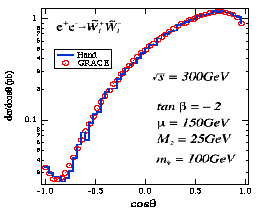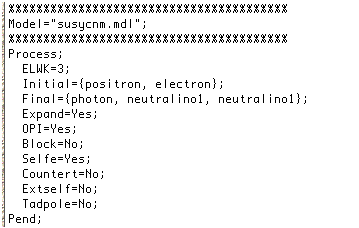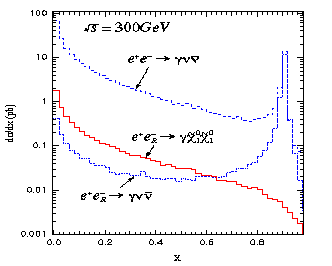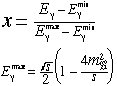# Automatic Calculation of Supersymmetric Amplitudes

## T.Kaneko, H.Tanaka, M.Jimbo, T.Kon and Minami-Tateya Collaboration at KEK

#### This document was originally prepared for a talk by T.Kon at Moriond conference at Les-Arc, France (March, 1995)## 1 Introduction

It should be emphasised that there are needs for automatic computation programs of Feynman amlitudes in the high energy particle phenomenology. Reasons are as follows. First, the present and future colliders could achieve the very high scattering energy, so processes with many body final states will become more important. Second, in almost known models, especially in SUSY models, many kinds of particles are included, so amplitudes with many virtual states will contribute to the physical processes. These two facts force us to calculate large number of Feynman diagrams. For example, number of Feynman diagrams for a 2 to 4 body process at e+e- colliders ; e+e- to e+e- plus two neutralinos, (this process will be important when the selectron is too heavy to be produced even singly at TeV linear colliders) are 7 handred 4 (!) and they are too many for us to calculate with ordinary methods : it must be tiresome and easy to mistake. Therefore, tools for automatic calculation will be needed for us. (Note that this example contains all contributions such as the electron-Higgs couplings and Goldstone boson couplings.)

There are several codes for automatic calculation of Feynman amplitudes
• 1. CompHep (Moscow)
• 3. FeynArts/FeynCalc (Wurzburg)
• 4. GRACE (Minami-Tateya Collab.(KEK))
It is known that they work very well for the Standard Model calculations. It should be mentoned that we have collaboration with LAPP (Annecy) in using GRACE for LEPII and NLC physics and Moscow group in cross checking between CompHep and GRACE system.
Here I will report a recent progress, i.e., The GRACE system becomes to be applicable for the calculation of the Supersymmetric (SUSY) processes.

## 2 GRACE for SUSY

Essentially, new features in the GRACE system are in following two points. 1)We can handle "Majorana" fermions such as the gluino and neutralinos, and 2)Particle mixings in the MSSM, neutralino and chargino mixings, are included.

Here I should clarify "what can GRACE do?" It can give us
• Graph generation
• Fortran code genetation
• (and also REDUCE and Form code generation)
• Phase space integration
• Event generation
"WE CAN GET CROSS SECTION AND GENERATED EVENTS AUTOMATICALLY!" It is important that the generated codes describe helicity amplitudes in the form with general covariant gauge. So we can check the gauge invariance of amplitudes numerically. And also we can freely choose polarization of initial and final state particles.

Now we should know "How to use GRACE?" These are steps for us to use GRACE.
• select a process
• select a model
• input model parameters
• run GRACE
• grc --> graph generation
• grcfort --> Fortran code generation
• integ --> phase space integration
I will show you these steps concretely in the next section.

## 3 Physical processes

In this section I will discuss some physical application of our system. Here are listed some elementary processes for checking the program.
• e-e- --> selectron- selectron-
• e-e+ --> selectron- selectron+
• e+e- --> chargino+ chargino-
• e+e- --> photino photino
• gamma gamma --> selectron+ selectron-
• gamma gamma --> chargino+ chargino-
• e+e- --> positron selectron photino
• e+e- --> photino photino photon
• e+e- --> neutralino1 neutralino1 photon
Now I will show some neumerical results.
For the MSSM processes, here is an example.This is the chargino angular distribution in the process
e+e- --> chargino+ chargino-.
Result by GRACE (red circle) and that by hand (blue line) are in agreement.

Next I will present another example for the MSSM in detail. This is a 2 to 3 body process,
e+e- --> photon neutralino1 neutralino1.
As you know, if the lightest neutralino is the LSP, it will escape the detector. So the signature of this process is very simple, single photon events.
In order to calculate this process, first, you should make the process file.All things you must do are to select the model, to select the order of coupling, and to define the initial and final particles. After running GRC, you can get these Feynman diagrams. If you like it, you can use it for your publication! Subsequently, run the sub-programs, GRCFORT and INTEG. Then you can get the output file, which consists of three parts. The first part describes input and output SUSY parameters, such as masses and mixing angles of gauginos. You can find the total cross section and some physical distributions in the second part and the third part on the file, respectively. In this example, the total cross section is about 0.11pb.
It is easy to retouch the histgram written in the output file as like this.The parameter x defined by this formularepresents essentially the scattering energy of the photon. Here we also plotted the background process, e+e- --> photon neutrino neutrino, which is also calculated by GRACE. Note, in our example, the LSP is almost the Bino, which is a good candidate for dark matter particle. We can see that the background is much larger than the SUSY process for unpolarized initial beams. But, fortunately, if we take the right-handed initial electron, the background can be suppressed and the signal could be extracted at the low energy region.

## 4 Summary

In summary, we have developed
"code for automatic calculation of Feynman amplitudes in SUSY models"
This is working well and could be a powerful tool for high energy particle phenomenology.
Finally, let me give you an announcement ; you can get GRACE for Standard model via anonymous ftp :
ftp.kek.jp/kek/minami/grace
(SUSY version will be available soon!)